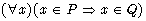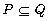5.4.2 Example of Actually Using a Definition

Furthermore, here, we demonstrateand it does not mean that we demonstrated. There is a mathematical distance between these two. However, the system understands the fact that both of them are equivalent by writing TARSKI in the definitions of the environ section as explained in the third chapter. It means that this is defined as a predicate in the abstract file of TARSKI.
TARSKI is structured from the definition which is the basis of the Mizar library; therefore, when writing articles, we write TARSKI at the definitions of the environ section.